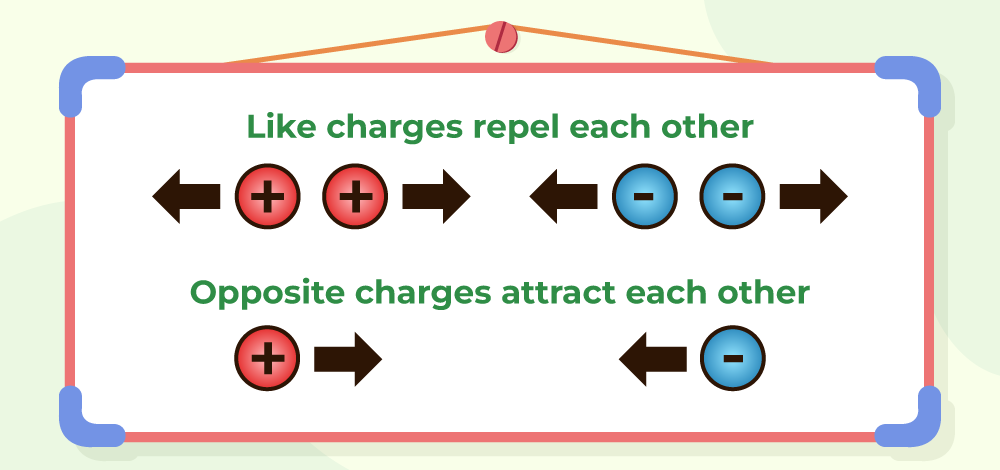GFG App
Open AppBrowser
Continue

Electric charge is the fundamental property of matter. Various properties are explained by the electric charges. So it is very important to note the unit of electric charge and other parameters of the electric charge. In this article, we will learn about electric charge its definition, and the units of electric charge in detail.

## Electric Charge

Electric charge is that property of matter which causes the matter to experience a certain force when placed in an electric field. Static charges produce Electric Field and when these charges start to move and become dynamic, they produce a magnetic field as well, moving charge is responsible for the production of electricity as well, to be precise, it is the movement of electrons that produces electricity.

### Electric Charge Formula

If the charge flowing inside a circuit producing electric current is needed to be calculated, the formula used for the same will be,

q = I × T

where,
I is the current flowing inside the circuit
T is the time for which the current is flowing.

## SI Unit of Electric Charge

Electric Charge can be measured in various units and the SI unit for measuring Electric Charge is Coulomb, which is represented by ‘C’. The value present of a single charge is 1.6 × 10-19C i.e. both proton and electron have a charge equal to the magnitude 1.6 × 10-19C

One coulomb is defined as the amount of charge that passes through an electrical conductor in one second which carries one-ampere current.

### Other Electric Charge Units

Electric Charge is measured in various units some of the other units of electric charge are,

### Other Units of Electrical

Electrical properties are widely used in science and some of the units that are used to measure various electrical properties are,

### Definie 1 Coulomb charge

1 Coulomb of charge can is defined as the amount of charge transferred in one second from a eire carrying current equal to qne Ampere.

## Types of Electric Charge

There are only two types of electric charges,

• Positive Charge
• Negative Charge

They both have the same amount of charge present on them, but with opposite signs.

Positive Charge

Positive charges are also known as Protons and the electric field lines come out of the positive charge. The charge present on a proton is +1.6 × 10-19C. If an object is positively charged, it can be concluded that the object has more protons than electrons.

Negative Charge

Negative charges are also known as electrons. The electric field lines come from infinity inside a negative charge. The amount of charge present on an electron is -1.6 × 10-19C. If an object is known to be negatively charged, that means that the object has more electrons than protons.

Note:

• Like charges repel each other
• Unlike charges attract each other## Coulomb’s Law

Coulomb’s Law is used to define the Force between two charges. Two charges can either have a force of attraction or a force of repulsion between them.

Let’s say that there are two charges q1, and q2 placed at a distance of ‘r’ between them, then the force between them will be defined as,where,is a ConstantHere, εo = 8.854× 10-12 C2/N-m2

The force between two charges q1 and q2 when they are placed in any other medium than Free Space,where,
ε is Absolute permittivity of the free space

If the ratio of the force in the free space and force in the medium is taken out, the relationship between absolute permittivity and relative permittivity (εr) is found,

ε/εo = εr

εr is Relative permittivity or Dielectric constant

Also, Check Coulomb’s Law

## Solved Examples on Electric Charges

Example 1: How many electrons are present in 1 Coulomb of charge?

Solution:

In a single electron, charge is equal to 1.6 × 10-19C

1.6× 10-19C = 1 electron

1C = 1/(1.6 × 10-19) electrons

1C = 6.25 × 1018 electrons

Example 2: How many Protons are present in 10 C of charge?

Solution:

According to the Quantization of charge,

Q = ne

Q = 10 C
n = number of protons
e = 1.6 × 10-19C

10 = n × 1.6× 10-19

n = 625 × 1017 protons

Example 3: In a system, the charges present are +5C, -7C, +1C, +9C, -15C. What is the total charge on the system?

Solution:

According to the Additivity property of Electric charges,

Q = q1+ q2+ q3+ q4+ q5

Q = +5C + (-7C) + 1C+ 9C+ (-15C)

Q = -7C

Example 4: Two charges +5C and +3C are placed at 1 meter apart in Free Space. Find the Force acting on them, and state the type of force as well.

Solution:

Force acting on +5C and +3C in free space is given by,Since, the charge +5C and +3C are alike in nature, i.e. they are both positive, hence, the force acting between them is the Repulsive Force.

## FAQs on Electric Charge

### Question 1: Define electric charge.

Electric charge is the fundamental property of the particles which is responsible for the elctromagnetic properties of the elements.

### Question 2: What are the types of electric charges?

Two types of electric charges are,

• Positive charge
• Negative charge

### Question 3: What is the SI unit of Electric Charge?

Electric Charge is measured in various units and the SI unit of electric charge is Coulomb.

### Question 4: What is the Formula to find the Electric Charge?

The Formula to find the Electric Charge is,

Q = I×t

where,
I is the current
t is the time taken